# Status Bar

 SysCAD in Edit Mode: Running in Steady State: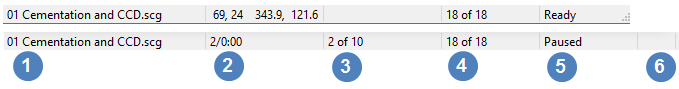Running Dynamic Simulation: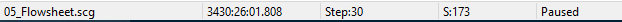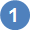displays the active window, in this case the Graphics Window.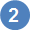displays different information depending on the current operation. if SysCAD is stopped and the mouse cursor is pointed on a Graphics Window, the number displayed is the position of the pointer. if SysCAD is running a Steady State simulation, it shows the number of iterations executed and time taken. For example "70/0:01.898" means the simulation has gone through 70 iterations and spent 1.898 seconds so far to solve the model. These values correspond to tags PlantModel.Stats.Iterations and PlantModel.Stats.SolveTime. if SysCAD is running a dynamic simulation, the number shown will represent the time span for which this project has been simulated.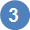Display different information depending on SysCAD solver mode. Steady State simulation: Shows the maximum error for convergence. This correspond to the value shown in Solver Status window. When the Max Error value is < 1.0 all the Criteria are considered converged (model is "solved"). The number of significant digits is based on the global relative tolerance specified. This value corresponds to tag \$Solver.Convergence.Error.[Worst].Max. Dynamic Simulation: Shows the step size.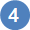Display different information depending on SysCAD solver mode. Steady State simulation: Shows Number of criteria solved. For example "7 of 10" means 7 of the 10 criteria for convergence have been met. These values correspond to Solver Setting - Convergence tags \$Solver.Convergence.Criteria.Active and \$Solver.Convergence.Criteria.Done. Dynamic Simulation: Shows the total number of steps executed, for example "S:52". If there are any time steps that did not converge, an additional count of Bad steps is given. For example "S:52 B:2" means that 2 of the 52 steps did not converge within the maximum number of allowed iterations. If more than one iteration is required to converge recycles within the current time step, then a count of the number of iterations for this step is shown. For example "S:52 (I:7)" or "S:52 B:2 (I:20)". These values correspond to Solver Setting - Convergence tags \$Solver.Convergence.Steps, \$Solver.Convergence.Steps.Bad and \$Solver.Convergence.Iterations. If the current step is a "bad" step and did not converge, that the value for "I:" will equal the maximum number of iterations allowed per step as specified in tag \$Solver.Convergence.MaxIters.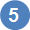Display the SysCAD State. See Plant Model - States - AppState and AppState.Detail for full list of states. These states are also available as special tags for OPC.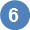If script commands are currently being executed, an additional pane showing the number of script commands to be executed will be shown.